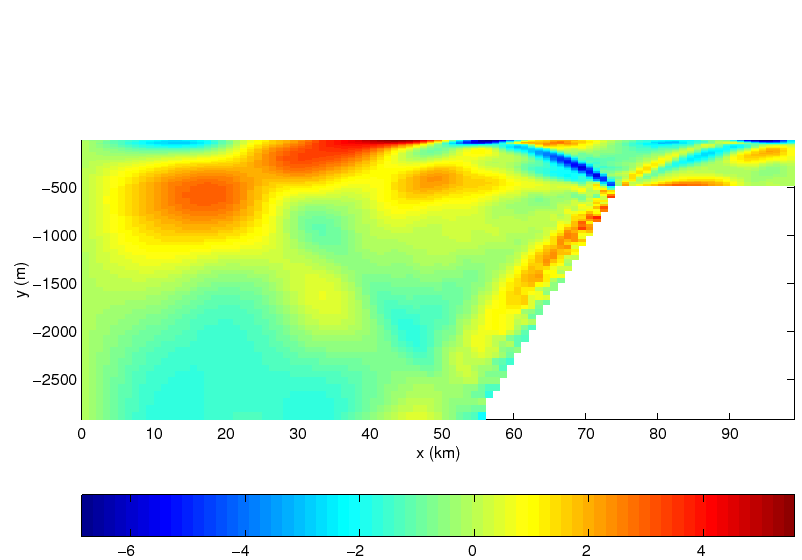Next: Parameter listing in suntans.dat Up: Internal waves Previous: Boundary conditions   Contents

### Running the test

The test case is run at the command line with the command

```make test
```
which runs the simulation for 3000 time steps (`suntans.dat: nsteps = 3000`) with a time step size of 29.808 s (`suntans.dat: dt = 29.808`) for a total of 2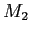tidal periods. This test case uses the sponge layer which is hardwired into SUNTANS. The parameters are set to `sponge_distance = 5000` and `sponge_decay = 7200` (for details see Section 7).

The results can be viewed with the `sunplot` gui from the main source directory with

```./sunplot --datadir=examples/iwaves/data
```
This brings up a planview of the one-dimensional grid of equilateral triangles. To display the profile plot of the results, press the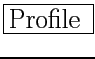button with the middle mouse button and then the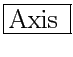button with the left mouse button so that the axes fill the plot window. This will display the salinity (density) field at the first time step, as shown in Figure 15. Plotting the u-velocity at data steps 11 and 21 will display the horizontal velocity field after one and two periods of forcing, respectively, as shown in Figures 16 and 17. Note that even with the use of the sponge layer, some internal wave energy is reflected back from the left boundary and affects the internal wave field shown in Figure 17. In addition to using `sunplot`, printouts for these plots can be obtained with the `plotslice.m` m-file which can be downloaded from

 0.75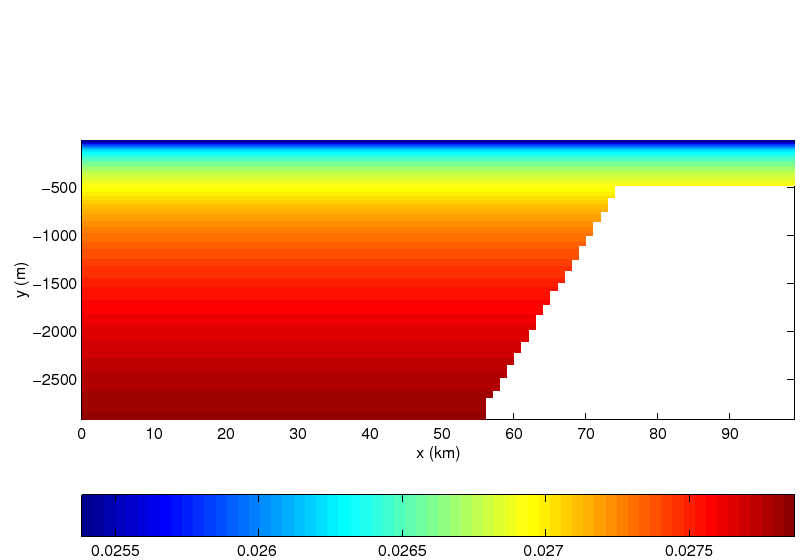0.75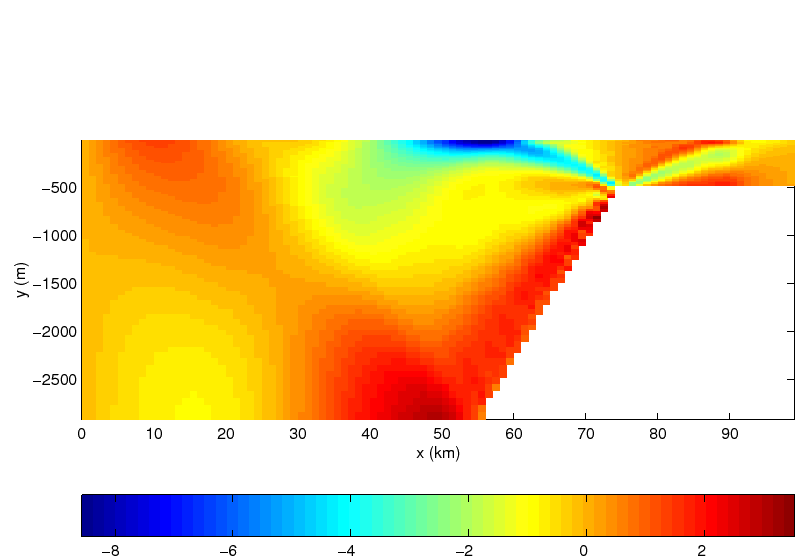0.75